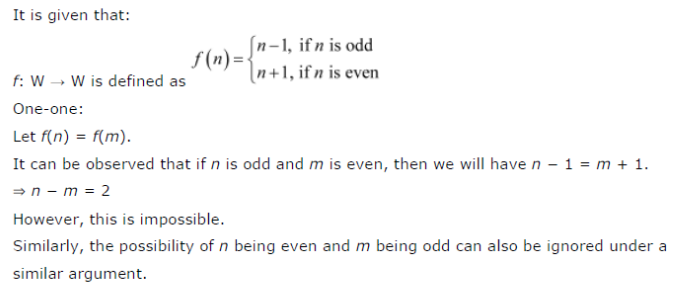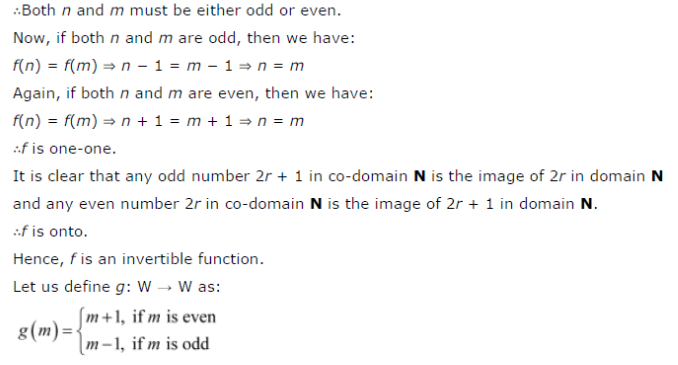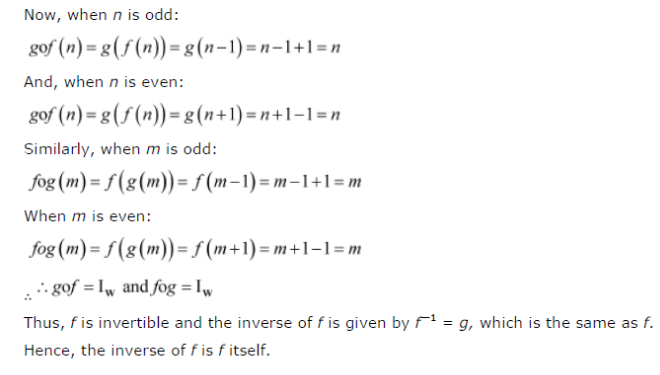# Let f : W → W be defined as f(n) = n - 1 if f is odd and f(n) = n + 1 if n is even

Let f : W → W be defined as f(n) = n - 1 if f is odd and f(n) = n + 1 if n is even. Show that f is invertible. Find the inverse of f Here, W is the set of all whole numbers.